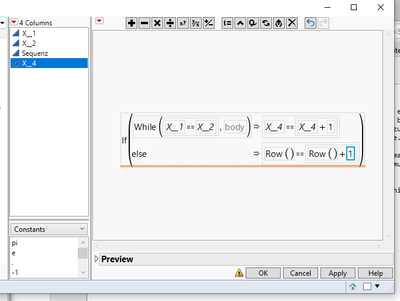Choose Language Hide Translation Bar
Highlighted

## formulaHello,

I want to  calculate from a file the length of the successive series of each individual.

How can i calculate this in JMP Pro with the formula?

By hand i have already entered a few sequences in the Column Sequenz.

X_4 =Sequenz

Thanks.

1 ACCEPTED SOLUTION

Accepted Solutions
Highlighted

## Re: formula

All of the information about the functions and statements in the script in question can be found in the Scripting Guide available under the Help pull down menu

Help==>JMP Documentation Library                Scripting Guide

I hope the following helps in the understanding of the formula

``````// The entire script will be run for each row in the
// in the data table

// On row 1 set default values
If( Row() == 1,
value = 1;
counter = 1;
);

// If the value of X__1 has changed check to see if
// more than one row has this new value of X__1
If( :X__1 != Lag( :X__1 ),
counter = 1;
While(
:X__1 == :X__1[Row() + counter]
|
Row() + counter == N Rows( Current Data Table() ),

counter
++);
value = counter;
,
// If this is not a row that has a new value of X__1
// then set the value to a missing value
value = .
);
// A JMP formula always takes the results of the last item
// processed, and makes that the value for the formula
// columns current row so by placing the variable that
// holds that value as the last item processed in the
// script, we ensure the correct value is placed into
// the data table
value;``````
Jim
3 REPLIES 3
Highlighted

## Re: formula

Here is the formula I came up with, that seems to work.

``````If( Row() == 1,
value = 1;
counter = 1;
);
If( :X__1 != Lag( :X__1 ),
counter = 1;
While( :X__1 == :X__1[Row() + counter] | Row() + counter == N Rows( Current Data Table() ), counter++ );
value = counter;
,
value = .
);
value;``````
Jim
Highlighted

## Re: formula

Hello,

this works great!

Could you please explain to me what this means.

If( :X__1 != Lag( :X__1 ),

...
counter = 1; While( :X__1 == :X__1[Row() + counter] | Row() + counter == N Rows( Current Data Table() ), counter++ );
value = counter;
,
value = .
);
value;

Thanks a lot!

Highlighted

## Re: formula

All of the information about the functions and statements in the script in question can be found in the Scripting Guide available under the Help pull down menu

Help==>JMP Documentation Library                Scripting Guide

I hope the following helps in the understanding of the formula

``````// The entire script will be run for each row in the
// in the data table

// On row 1 set default values
If( Row() == 1,
value = 1;
counter = 1;
);

// If the value of X__1 has changed check to see if
// more than one row has this new value of X__1
If( :X__1 != Lag( :X__1 ),
counter = 1;
While(
:X__1 == :X__1[Row() + counter]
|
Row() + counter == N Rows( Current Data Table() ),

counter
++);
value = counter;
,
// If this is not a row that has a new value of X__1
// then set the value to a missing value
value = .
);
// A JMP formula always takes the results of the last item
// processed, and makes that the value for the formula
// columns current row so by placing the variable that
// holds that value as the last item processed in the
// script, we ensure the correct value is placed into
// the data table
value;``````
Jim
Article Labels

There are no labels assigned to this post.